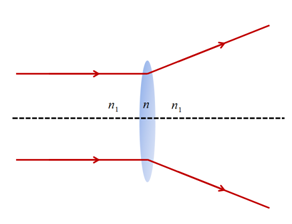# Ray Optics MCQs for NEET

In optics, light is considered to be a ray that travels in a straight line. Following are a few important terms that we often deal with while studying light:

• The phenomenon of the light returning to the same medium from which it started after striking a surface is known as a reflection of light.
• There are two laws of reflection as follows

(a) The angle of incidence is equal to the angle of reflection

(b) The incident ray, the reflected ray and the normal to the reflecting surface all lie in the same plane.

• When a ray of light travelling from a denser medium to a rarer medium at an angle greater than the critical angle, it is completely reflected back to the denser medium. This phenomenon is called the total internal reflection.
• Power of lens is defined as the ability of the lens to converge or diverge the rays of light.
• The phenomenon of splitting of light into its component colours is known as dispersion.

### Ray Optics: Question and Answer

1. What is the distance between two convex lenses LA and LB with focal lengths FA and FB?

1. FA+FB
2. FA-FB
3. FA
4. FB

2. If a medium has a critical angle for total internal reflection from the medium to vacuum as 300, what is the velocity of light in the medium?

1. 0.5 × 108 m/s
2. 3 × 108 m/s
3. 1.5 × 108 m/s
4. 0.2 × 108 m/s

Answer: (c) 1.5 × 108 m/s

3. The combination of which of the following processes results in the formation of a rainbow?

1. Dispersion and Total Internal Reflection
2. Dispersion and Absorption
3. Refraction and Scattering
4. Dispersion and Focussing

Answer: (a) Dispersion and Total Internal Reflection

4. What is the focal length of a double concave lens with a radius of curvature 20 cm, if the refractive index of the glass with respect to the air is 5/3?

1. 20 cm
2. -20 cm
3. 15 cm
4. -15 cm

5. A diverging lens of power 65 D is combined with a convex lens of focal length, f = 20 cm. What are the power and focal length of the combination?

1. 5 D, 33.7 cm
2. 5 D, 66.7 cm
3. 1.5 D, 33.7 cm
4. -1.5 D, 66.7 cm

Answer: (b) 5 D, 66.7 cm

6. When a thin convex lens of glass 5D is immersed in a liquid, it behaves as a divergent lens of focal length 100 cm. What is the refractive index of the liquid?

1. 5/3

7. The magnifying power of an astronomical telescope in normal adjustment is 100. What is the focal length of the objectives and eyepiece of the distance between them is 101 cm?

1. 1 cm and 10 cm respectively
2. 1 cm and 100 cm respectively
3. 10 cm and 1 cm respectively
4. 100 cm and 1 cm respectively

Answer: (d) 100 cm and 1 cm respectively

8. If an equiconvex lens of focal length f and power P is cut into half in thickness, what is the focal length and power of each half?

1. Zero
2. f/2
3. f
4. 2f

9. A prism has an angle 600 and refractive index √2, what is the angle of minimum deviation?

1. 900
2. 600
3. 450
4. 300

10. What is the correct relationship between refractive indices n,n1 and n2 if the behaviour of light is as shown in the figure?1. n1>n
2. n1<n
3. n1=n
4. None of the above